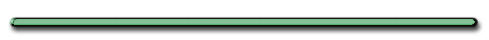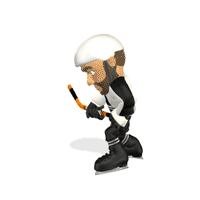2nd Law - Relationship between F, m, a

Unbalanced forces
cause objects to accelerate:

ΣFnet = ma

Fnet = ResultantEx) Mass M below is lifted up with an acceleration a. Find the tension in the string TΣFnet = ma

(up positive)T - mg = ma

T = ma + mg

String Pulled up

T = m(g + a)

String Acelerated down?

T = m(g - a)

Two masses are connected by a string.

Find tension force on top and bottom rope.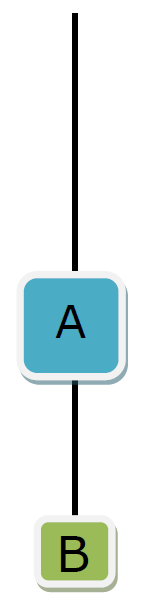Ex 1) Two masses are connected by a string and remain at rest.

Find tension force on top and bottom rope.SF = 0

Top String Tension Force

TA - mag - mbg = 0

TA = mag + mbg

Bottom String Tension Force

TB - mbg = 0

TB = mBg

Ex 2) Find the tension of top and bottom string if objects accelerated upward

Free body diagram B (easier)

Sketch all forces on AForce of tension on B (easier)

Free body diagram B

Sketch all forces on B

SF = mBa

TB - mBg = mBa

TB = mBa + mBg

= (force of accel. of B + weight of B)

Sketch all forces on A

SF = mAa

TA - TB - mAg = mAa

TA = mAa + mAg + TB

Since TB = mBa + mBg

TA = mAa + mAg +mBa+mBg)

TA=(mAa+mBa)+(mBg+mAg)

= (force of accel) + (weights)

Giancoli p. 98) 8, 25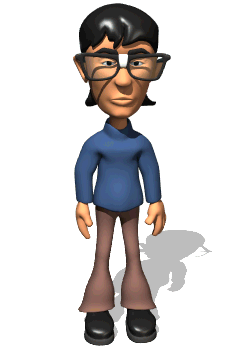"Which cart is slowing down as it travels to  the right?"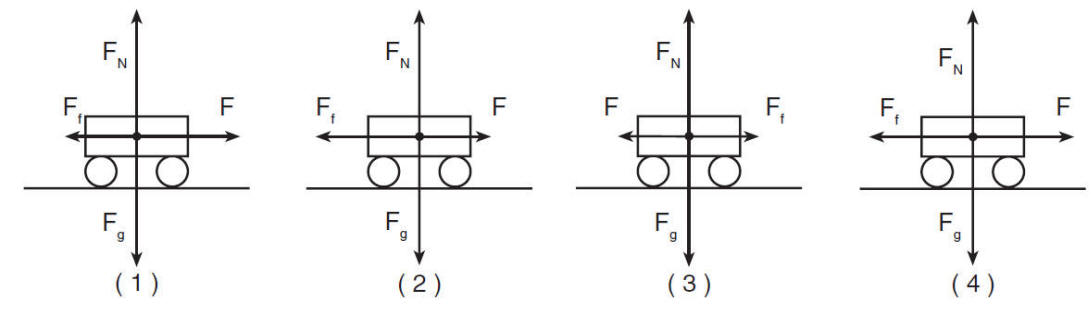Choice 2Check out this